# RequestArtemis landers

#### Gargantua2024

##### The Desktop Orbinaut
.. Can you post the scn. I will make the adapter a cfg vessel and add it
SCN and MS2015.ini attached below...I also modified the BaseSpaceX\Canaveral.cfg so that Pad 39A and B can coexist with each other during simulation

#### Attachments

• SLS_ALPACA.zip
6.9 KB · Views: 31

#### Gargantua2024

##### The Desktop Orbinaut
SLS (Block 1B) and ALPACA test 2....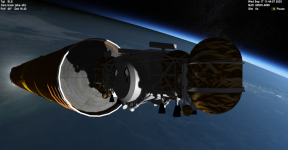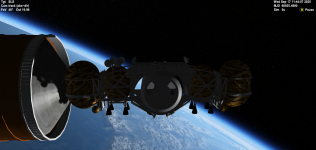Lowered the droptanks so that it is centered inside the rocket as rendered by Dynetics:
Code:
MESH_1_NAME = dyneticstANK
MESH_1_POS = 6.1 0.1 0
MESH_1_DIR = 0 0 1
MESH_1_ROT = 0 1 0
MESH_1_VIS = 1

MESH_2_NAME = dyneticstANK1
MESH_2_POS = -6.1 0.1 0
MESH_2_DIR = 0 0 1
MESH_2_ROT = 0 1 0
MESH_2_VIS = 1
Code:
EVENT_0_ID = 0
EVENT_0_NAME = Jettison 1
EVENT_0_TYPE = 1
EVENT_0_TRIGGERTYPE = 2
EVENT_0_REPEAT = 0
EVENT_0_KEY = 36
EVENT_0_KEYMOD = 0
EVENT_0_SPAWNEDCLASS = Stage
EVENT_0_SPAWNEDNAME = DyneticsTANK
EVENT_0_SPAWNEDOFS = 6.1 0.1 0
EVENT_0_SPAWNEDVEL = 2 -0.5 0
EVENT_0_SPAWNEDROTVEL = 0 0 0
EVENT_0_SPAWNDELMESH = 1

EVENT_1_ID = 1
EVENT_1_NAME = Jettison 2
EVENT_1_TYPE = 1
EVENT_1_TRIGGERTYPE = 2
EVENT_1_REPEAT = 0
EVENT_1_KEY = 36
EVENT_1_KEYMOD = 0
EVENT_1_SPAWNEDCLASS = Stage
EVENT_1_SPAWNEDNAME = DyneticsTANK1
EVENT_1_SPAWNEDOFS = -6.1 0.1 0
EVENT_1_SPAWNEDVEL = -2 -0.5 0
EVENT_1_SPAWNEDROTVEL = 0 0 0
EVENT_1_SPAWNDELMESH = 2
SLS_ALPACA.ini file slightly updated above

#### barrygolden

##### Active member
The guys are looking great. add stripes to the legs and the sunshields up while flying the ALPACA.

#### barrygolden

##### Active member
I did like the SLS launch and config looks great. legs need to be folded and it needs a space tug to place it into Lunar orbit. make note of the gold object docked to this early version of gateway. Its the OMV from the Shuttle days.
I still see this as the way to have the Gateway with 1 launch instead of 6 launches.

#### Gargantua2024

##### The Desktop Orbinaut
I still see this as the way to have the Gateway with 1 launch instead of 6 launches.
If they need to launch Gateway in a single SLS, then maybe NASA should've selected the Skylab II proposal insteadIf I recall correctly, there's an existing addon for this station already, but built for O2010

#### barrygolden

##### Active member
yes do you have a link to that. can you do and SLS/Orion scn as well ? gattis did a modified octopus we called the docking module and a modified Orion called Eagle/Talon. He did a super job with both you can see this could be the start of a single launch Gateway with multiple docking ports. The Eagle has extra tankage to refuel of replace the drop tanks and the White tank could be a cargo bay to bring up new surface tools or supplies to be added to the ALPACA

#### barrygolden

##### Active member
The Apollo guys knew even though they had a open checkbook to land on the Moon that cost drove there decisions. these new NASA guys have forgot that. It will be hard to see this flying as is." See station Freedom." However this is on a decadal survey so the funding is set for ten years but NASA never has done what they say they can do with out massive cost over runs with the exception of Skylab

#### Gargantua2024

##### The Desktop Orbinaut
yes do you have a link to that.
Skylab II is included in this early version of the SLS addon also made by gattispilot

can you do and SLS/Orion scn as well?
Yep I could do an SLS/Orion scenario as well, but I think it already exists in the Space Launch System addon, albeit it is still named as Exploration Missions instead of Artemis

gattis did a modified octopus we called the docking module and a modified Orion called Eagle/Talon.
Looks like the Eagle/Talon resembles the current iteration of the Gateway's HALO module

•gattispilot

#### Gargantua2024

##### The Desktop Orbinaut
I've been thinking that we should consider francisdrake's Project MAV - The Frisbee of Mars as the Boeing HLS, and the Altair LSAM as the Vivace HLS.....with that, we have now four HLS proposals out of five total

•barrygolden

#### gattispilot

This is problem of making things in the future. They change.

Here is going to be another issue. We are going to have different versions. different crew,.....

we will get this.. The other guy will be the same except for the red/white bands

#### Attachments

25.5 KB · Views: 6
Last edited:
•barrygolden

#### barrygolden

##### Active member
Looks pretty spot on, looking to see it in the ALPACA

What does the SLS adapter do ?

Last edited:

#### gattispilotIt has red/green beacons. They just did not show in the snapshot.

I need to play around with the setting of the guys. May not want to see internal just external? Because it blocks the view and mfd,..... I just moved the mesh. The guy would have a backpack one

close up of the guy inside:Last edited:
••Interceptor, Gargantua2024 and barrygolden

#### Gargantua2024

##### The Desktop Orbinaut
SLS (Block 1B) and ALPACA test 3...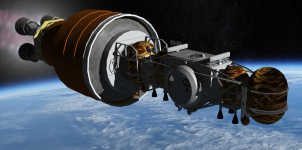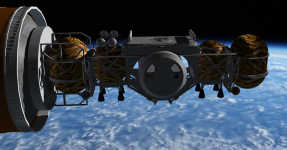New adapter mesh now included...still use the same SCN file, no need to modify it except for the .ini below:
Code:
[MISC]
cog=25.000
GNC_Debug=0
Telemetry=0
Focus=1
Thrust_real_pos=0
Vertical_Angle=0.000

[TEXTURE_LIST]
TEX_1=Exhaust_atsme
TEX_2=SLS_2016\SSME_Exhaust
TEX_3=SLS_2016\SLS_SRB_contrail

[PARTICLESTREAM_1]
Name=SRB_exhaust
SRCSIZE=3
SRCRATE=12
V0=50
GROWTHRATE=3
ATMSLOWDOWN=0.3
LTYPE=DIFFUSE
LEVELMAP=LVL_PSQRT
LMIN=0
LMAX=1
ATMSMAP=ATM_PLOG
AMIN=1e-6
AMAX=1
Tex=SLS_2016\SLS_SRB_contrail
GROWFACTOR_RATE=6
GROWFACTOR_SIZE=0.2

[PARTICLESTREAM_2]
Name=SRB_exhaust_2
SRCSIZE=3
SRCRATE=12
V0=30
GROWTHRATE=3
ATMSLOWDOWN=0.3
LTYPE=EMISSIVE
LEVELMAP=LVL_PSQRT
LMIN=0
LMAX=1
ATMSMAP=ATM_PLOG
AMIN=1e-6
AMAX=1
TEX=contrail2
GROWFACTOR_RATE=6
GROWFACTOR_SIZE=0.2

[PARTICLESTREAM_3]
Name=Launchfx
Srcsize=10.000
Srcrate=30.000
V0=170.000
Growthrate=10.000
Atmslowdown=0.300
Ltype=DIFFUSE
Levelmap=LVL_PSQRT
Lmin=0.8
Lmax=1.000
Atmsmap=ATM_PLOG
Amin=1e-006
Amax=1.000
Tex=SLS_2016\SLS_SRB_contrail

[PARTICLESTREAM_4]
Name=vent
amin =1e-006
amax =1.000
atmslowdown =4.000
growthrate =1.900
lmax =0.500
lmin =0
srcrate =8.000
srcsize =0.400
v0 =0.500
ltype =EMISSIVE
atmsmap =ATM_PLOG
levelmap =LVL_PSQRT
tex =contrail
GrowFactor_size=20.000
GrowFactor_rate=8.000

[PARTICLESTREAM_5]
NAME=mach
SRCSIZE=12
SRCRATE=20
V0=50.1
GROWTHRATE=10
ATMSLOWDOWN=0
LTYPE=EMISSIVE
LEVELMAP=LVL_PSQRT
LMIN=0
LMAX=4
ATMSMAP=ATM_PLOG
AMIN=0.7e-7
AMAX=1
TEX= contrail

[FX_LAUNCH]
N=2
Height=10.000
Angle=90.000
Distance=0.000
CutoffAltitude=800.000
Pstream1=launchfx
Pstream2=launchfx2

[FX_VENT]
pstream=vent
off_1=(4.200,-1.000,30.000)
dir_1=(1.000,5.000,0.000)
time_fin_1=-5.000
off_2=(1.000,-4.200,15.000)
dir_2=(5.000,1.000,0.000)
time_fin_2=-5.000

[FX_MACH]
pstream=mach
mach_min=0.900
mach_max=1.300
off_1=(-1.000,0.000,18.000)
off_2=(1.000,0.000,18.000)
off_3=(-5.000,0.000,5.000)
dir=(0.000,0.000,-1.000)
Off_4=(5.000,0.000,5.000)
Off_5=(0.000,0.000,30.000)

[BOOSTER_1]
N=1
MeshName=SLS_2016\SLSSRBNOCHECKERBOARD
Height=53.900
Diameter=3.710
EmptyMass=86363.600
FuelMass=622727.300
Thrust=16363630.640
angle=0.000
off=(6.300,0.000,-0.300)
BurnTime=124.000
eng_1=(0.000,0.000,-28.000)
eng_diameter=0.010
ENG_PSTREAM1=srb_exhaust
Speed=(15.000,-4.000,0.000)
Rot_speed=(-0.200,-0.400,0.000)
Eng_tex=
Eng_pstream1=SRB_exhaust
Eng_pstream2=srb_exhaust_2
BurnDelay=0.000
Expbolts_pos=(0,1.000,19.000)
Expbolts_pstream=exbolt
Expbolts_anticipation=1

[BOOSTER_2]
N=1
Angle=0.000
Meshname=SLS_2016\SLSSRB_1NOCHECKERBOARD
Off=(-6.300,0.000,-0.300)
Height=53.900
Diameter=3.710
Thrust=16363630.640
EmptyMass=86363.600
FuelMass=622727.300
Burntime=124.000
Eng_diameter=0.010
Eng_tex=
Eng_pstream1=srb_exhaust
Eng_pstream2=srb_exhaust_2
Speed=(-15.000,-4.000,0.000)
Rot_speed=(-0.200,0.400,0.000)
Eng_1=(0.000,0.000,-28.000)
BurnDelay=0.000
Expbolts_pos=(-6.450,1.000,25.000)
Expbolts_pstream=exbolt
Expbolts_anticipation=1

[STAGE_1]
Height=63.720
Diameter=8.400
EmptyMass=85275.392
FuelMass=979452.000
Thrust=9116000.375
BurnTime=476.000
off=(0.000,0.000,4.700)
MeshName=SLS_2016\SLSCORE_NOCHECKERBOARD
eng_1=(-2.300,2.300,-32.208)
eng_2=(-2.300,-2.300,-32.208)
eng_3=(2.300,-2.300,-32.208)
eng_4=(2.300,2.300,-32.208)
eng_diameter=2.400
PITCHTHRUST=14193633.100
YAWTHRUST=14193633.100
speed=(0.000,0.000,-2.000)
ENG_PSTREAM1=engdetail_2
battery=1.500
Reignitable=1
Eng_tex=SLS_2016\SSME_Exhaust
Eng_pstream2=engdetail
Eng_dir=(0.000,0.000,1.000)
Module=Stage
Rot_speed=(0.000,0.000,0.000)

[STAGE_2]
MeshName=SLS_2016\EUS_ORANGE3
Diameter=8.400
Height=17.700
EmptyMass=19000.000
FuelMass=109000.000
Thrust=440400.000
BurnTime=1350.000
ignite_delay=10.000
off=(0.000,0.000,44.70)
eng_1=(1.350,-1.350,-9.000)
eng_2=(1.350,1.350,-9.000)
eng_3=(-1.350,1.350,-9.000)
eng_4=(-1.350,-1.350,-9.000)
eng_diameter=1.700
PITCHTHRUST=2331995.000
YAWTHRUST=2331995.000
ullage_thrust=100000.000
ullage_N=4
ullage_angle=-22.500
ullage_pos=(4.200,0.000,-8.000)
ullage_dir=(0.200,0.000,-1.000)
ullage_tex=Exhaust_atsme
ullage_length=5.000
ullage_diameter=1.000
ullage_anticipation=3.000
ullage_overlap=1.000
ullage_rectfactor=3.000
reignitable=1
Eng_tex=SLS_2016\SSME_Exhaust
Eng_pstream1=
Eng_pstream2=
Eng_dir=(0.000,0.000,1.000)
Module=Stage
Speed=(0.000,0.000,0.000)
Rot_speed=(0.000,0.000,0.000)

[FAIRING]
N=2
MeshName=SLS_2016\BLOCK1BCARGOFAIR_1
Diameter=10.000
Height=31.350
angle=0.000
off=(0.000,0.000,44.750)
EMPTYMASS=10683.000
speed=(0.000,-7.500,0.000)
Module=Stage
Rot_Speed=(-0.3000,0.0300,0.000)

off=(0.0,0.0,44.6)
Height=1
Diameter=3.07
EmptyMass=1000

MeshName=dyneticstest2
off=(0.000,0.000,61.20)
Diameter=5.000
Height=8.000
MASS=40800.000
Module=VesselBuilder1\Dynetictest
Name=ALPACA
SPEED=(0.000,0.000,2.000)
RENDER=1
Rotation=(0.000,90.000,0.000)
Rot_speed=(0.000,0.000,0.000)
Live=1

Last edited:

#### barrygolden

##### Active member
Looks super !! I did see with the last SLS /ALPACA launch looked like room for your LER might fit and replace the cockpit.

Might look at the HGA's folding up as well

•gattispilot

#### Gargantua2024

##### The Desktop Orbinaut
Looks super !! I did see with the last SLS /ALPACA launch looked like room for your LER might fit and replace the cockpit.
That's part of the Dynetics design....intentionally replace the cabin with some other payloads for constructing Artemis Base Camp

#### gattispilot

Got the hga to bend.now the array tilts, rotates around the arm. And the arm rotates.

I guess we need to go with one design?

I can make the lander without the Habitat. and then add an attachment for what ever is needed.

#### Gargantua2024

##### The Desktop Orbinaut
I can make the lander without the Habitat. and then add an attachment for what ever is needed.
Sounds good....just need to add a new Reconfiguration for the cargo variant once the crew version of the addon is finalized since technically they have the same specs

•Interceptor and gattispilot

#### gattispilot

yes. We would get something like this:My fear is when the LER is dettach it will bounceI think we are close to a final version? Use the new crew guys and moveable hga, beacons.

#### Gargantua2024

##### The Desktop Orbinaut
yes. We would get something like this:My fear is when the LER is dettach it will bounceNow we do now know how to put the SEV-LER into space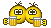I think we are close to a final version? Use the new crew guys and moveable hga, beacons.
I agree, I think we really are close to the final version....the only integral part left on the Dynetics plan (I think) is the intended launcher itself besides SLS...the Vulcan-Centaur

•barrygolden and gattispilot